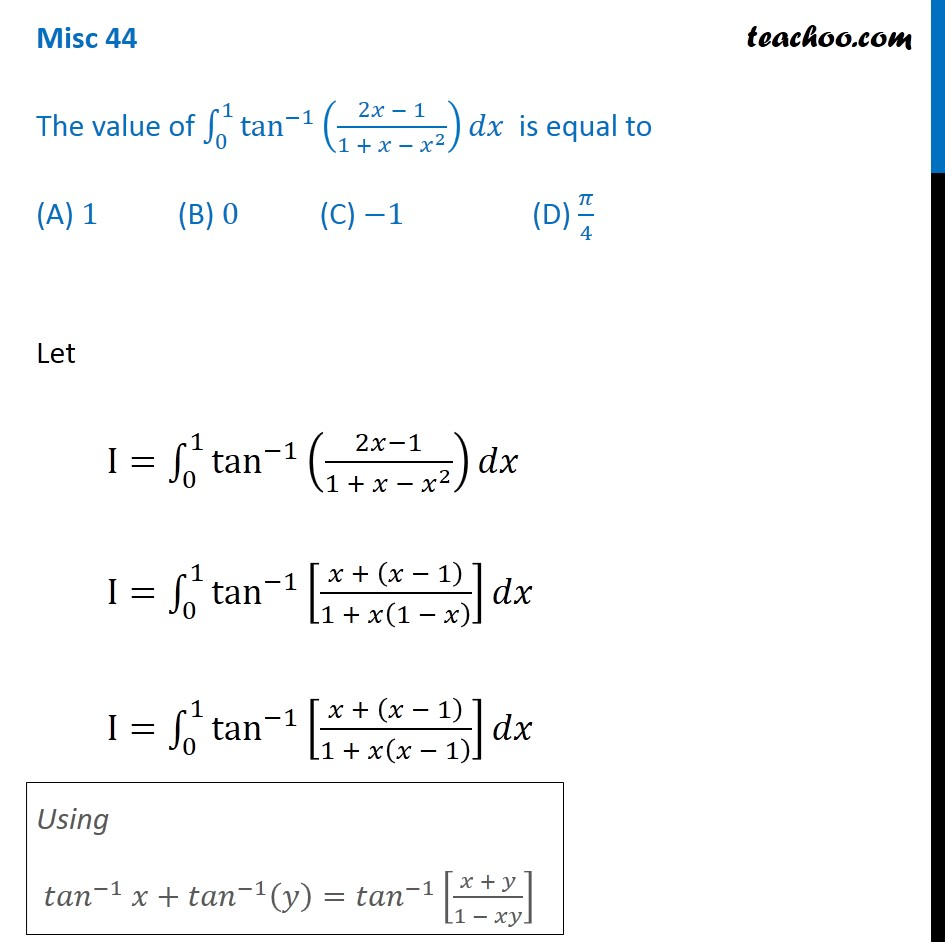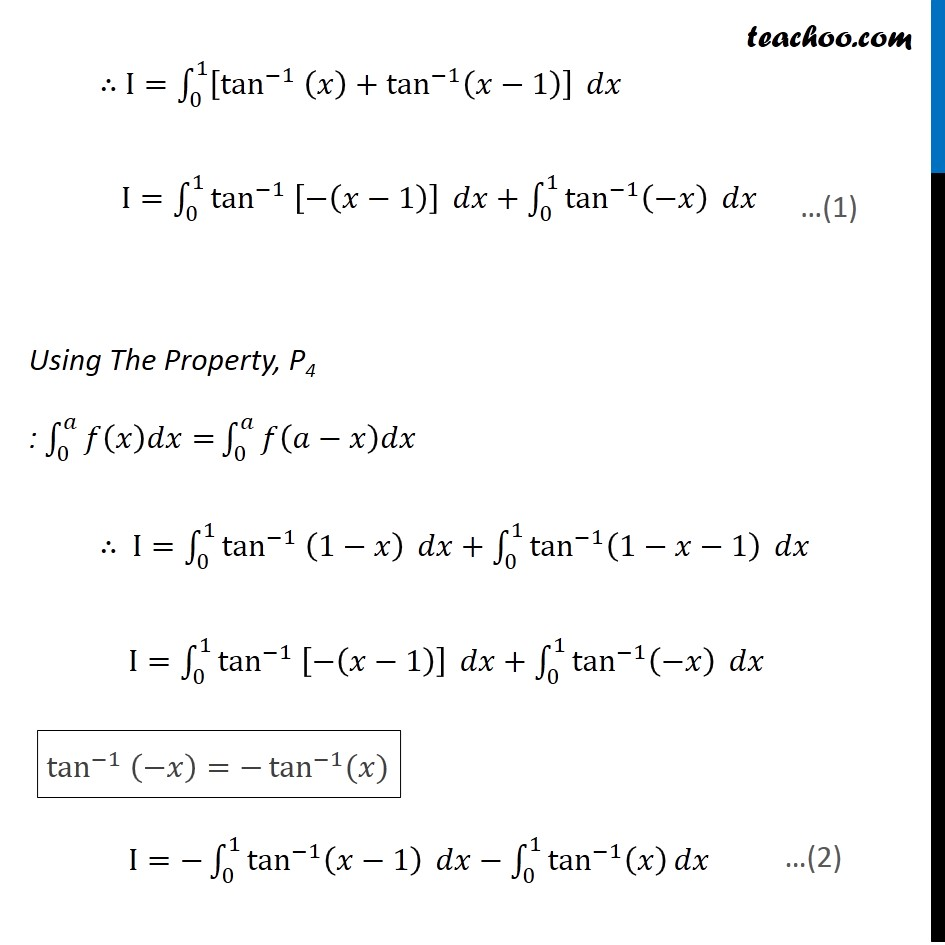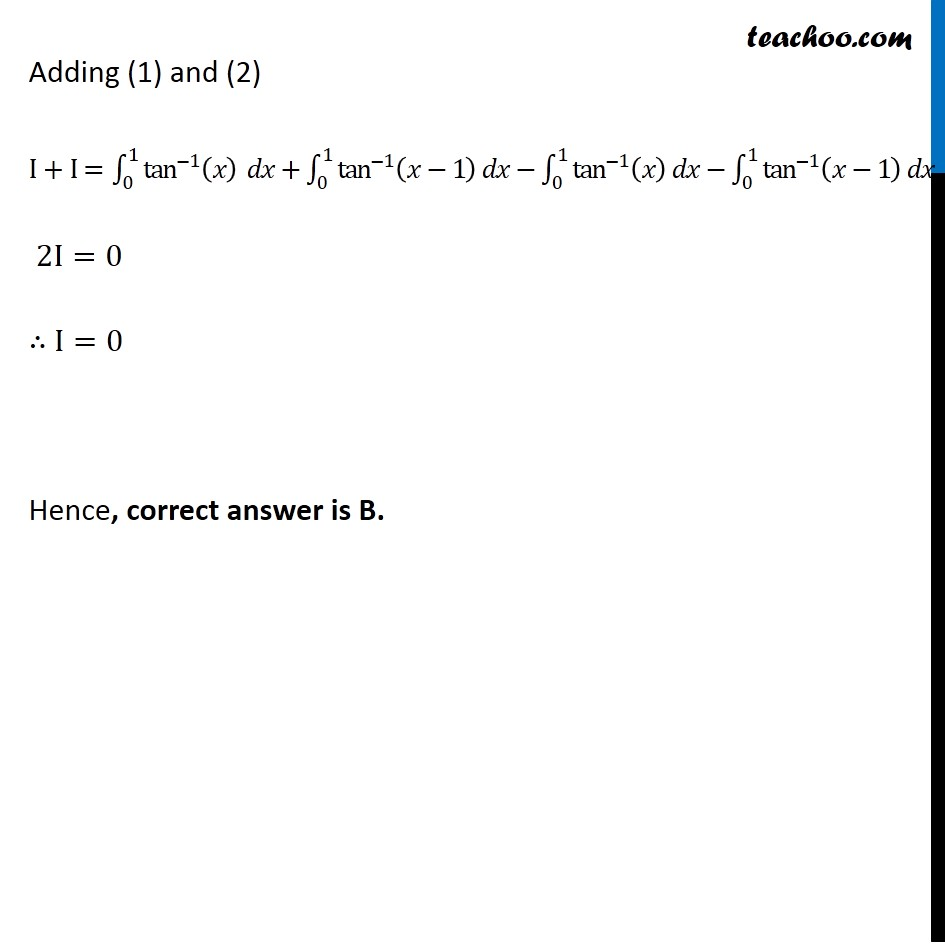Definite Integration by properties - P4

Chapter 7 Class 12 Integrals
Concept wiseLearn in your speed, with individual attention - Teachoo Maths 1-on-1 Class

### Transcript

Misc 44 The value of ∫_0^1▒tan^(−1)⁡((2𝑥 − 1)/(1 + 𝑥 − 𝑥^2 )) 𝑑𝑥 is equal to (A) 1 (B) 0 (C) −1 (D) 𝜋/4 Let I=∫_0^1▒tan^(−1)⁡((2𝑥−1)/(1 + 𝑥 − 𝑥^2 )) 𝑑𝑥 I=∫_0^1▒tan^(−1)⁡[(𝑥 + (𝑥 − 1))/(1 + 𝑥(1 − 𝑥) )] 𝑑𝑥 I=∫_0^1▒tan^(−1)⁡[(𝑥 + (𝑥 − 1))/(1 + 𝑥(𝑥 − 1) )] 𝑑𝑥 Using 〖𝑡𝑎𝑛〗^(−1) 𝑥+〖𝑡𝑎𝑛〗^(−1) (𝑦)=〖𝑡𝑎𝑛〗^(−1)⁡[(𝑥 + 𝑦)/(1 − 𝑥𝑦)] ∴ I=∫_0^1▒[tan^(−1) (𝑥)+tan^(−1) (𝑥−1)] 𝑑𝑥 I=∫_0^1▒〖tan^(−1) [−(𝑥−1)] 〗 𝑑𝑥+∫_0^1▒〖tan^(−1) (−𝑥) 〗 𝑑𝑥 Using The Property, P4 : ∫_0^𝑎▒〖𝑓(𝑥)𝑑𝑥=〗 ∫_0^𝑎▒𝑓(𝑎−𝑥)𝑑𝑥 ∴ I=∫_0^1▒〖tan^(−1) (1−𝑥) 〗 𝑑𝑥+∫_0^1▒〖tan^(−1) (1−𝑥−1) 〗 𝑑𝑥 I=∫_0^1▒〖tan^(−1) [−(𝑥−1)] 〗 𝑑𝑥+∫_0^1▒〖tan^(−1) (−𝑥) 〗 𝑑𝑥 I=−∫_0^1▒〖tan^(−1) (𝑥−1) 〗 𝑑𝑥−∫_0^1▒〖tan^(−1) (𝑥) 〗 𝑑𝑥 tan^(−1) (−𝑥)=−tan^(−1)⁡〖(𝑥)〗 Adding (1) and (2) I+I =∫_0^1▒〖tan^(−1) (𝑥) 〗 𝑑𝑥+∫_0^1▒〖tan^(−1) (𝑥−1) 〗 𝑑𝑥−∫_0^1▒〖tan^(−1) (𝑥) 〗 𝑑𝑥−∫_0^1▒〖tan^(−1) (𝑥−1) 〗 𝑑𝑥 2I=0 ∴ I=0 Hence, correct answer is B.